International
Tables for
Crystallography
Volume B
Reciprocal space
Edited by U. Shmueli

International Tables for Crystallography (2010). Vol. B, ch. 2.5, pp. 382-383

## Section 2.5.7.8. Ab initio 3D structure determination using computational methods

P. A. Penczekg

#### 2.5.7.8. Ab initio 3D structure determination using computational methods

| top | pdf |

The experiment-based methods of initial 3D structure determination (RCT and electron tomography) are quite powerful, but rather challenging to employ in practice. Particularly frustrating is the fact that a large volume of difficult-to-record tilt data have to be collected, even though they cannot be used for subsequent high-resolution work. Therefore, whenever possible, preference is given to computational methods in which 3D geometrical relations between particle projections are established using various mathematical approaches using only untilted data.

The most straightforward approach and historically the earliest is based on the central section theorem [equation (2.5.6.8)of Section 2.5.6]: because Fourier transforms of 2D projections of a 3D object are the central section of the 3D Fourier transform of this object, it is a straightforward consequence that Fourier transforms of any two projections intersect along a line, henceforth called a common line. (Two trivial exceptions are the case of projections in the same direction, in which their Fourier transforms coincide with possible differences in in-plane rotation, and the case of projections in opposite directions, in which they are mirror versions of each other.) This fact was originally used by Crowther, DeRosier & Klug (1970) to solve the structure of viruses with icosahedral (60-fold) symmetry. In this case, the Fourier transform of each projection intersects itself (or rather the symmetry-related copies of itself) 37 times with the exception of degenerate cases of projections in directions of one of three symmetry axes, in which cases the number of common lines is less. Thus, it is possible to find the orientation of a single projection with respect to the chosen system of symmetry axes.

For asymmetric objects a set of three projections that do not intersect along the same line (which would correspond to the single-axis tilt geometry) uniquely determine their respective orientations (with the exception of the overall rotation, which remains arbitrary, and the handedness of the solution, which remains undetermined). Indeed, three projections span three common lines, and each common line yields two angles: for each of the intersecting sections it is the angle between the x axis in the system of coordinates of this section and the common line in the plane of this section. Thus, we have a total of six angles. At the same time, by arbitrarily setting the orientation of the first projection in 3D space to three Eulerian angles equal to zero (or the corresponding rotation matrix R1 = I), we need to determine two rotation matrices R2 and R3 (or two sets of three Eulerian angles) for the remaining two projections, respectively. So, given six in-plane angles we have to find a solution for six Eulerian angles. Let the angle of the common line between the ith and jth projection in the plane of the ith projection be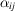and the corresponding unit vector in the plane of ith projection be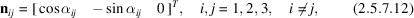where we added the third coordinate for convenience. The orientations of unit vectors nij in 3D space have to be related by rotation matrices; for example, vector n21 (the direction of the common line between the first and second projection in the plane of the second projection) should coincide with vector n12 (the direction of the same common line, but in the plane of the first projection) upon rotation by (unknown) matrix R2. All possible relations are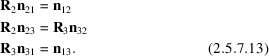Equations (2.5.7.13)have two solutions corresponding to two different hands of the molecule [for details see Farrow & Ottensmeyer (1992)].

The common-lines method works very well in the absence of noise. However, even a modest amount of noise can yield quite erroneous results or no results at all. The reason is that the solution of (2.5.7.13)is highly nonlinear with respect to the six given anglesand small errors in the location of peaks in cross-correlation functions can lead to quite large discrepancies from the correct solution. The main difficulty with the application of the common-lines method is that the analytical solution in the form of (2.5.7.13)exists only for three projections (Goncharov et al., 1987).

As a working approach to ab initio structure determination, the common-lines method has been implemented under the name of angular reconstitution in IMAGIC (van Heel, 1987a; van Heel et al., 1996). In order to reduce sensitivity to noise, the method is applied not to individual projection images, but to class averages resulting from the multireference alignment of input data (van Heel et al., 2000). In order to overcome the problem of the lack of solution for the larger than three number of projections, the user has to begin with selection of three judiciously chosen class averages, obtain the solution using (2.5.7.13)and subsequently include (angle) additional class averages using a brute-force approach, in which the Eulerian angles of the new projection are calculated using a similarity measure based on common lines with the already-angled set serving as a reference.

Some of the disadvantages of the angular reconstitution were addressed in the common-lines-based method for determining orientations for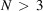particle projections simultaneously (Penczek et al., 1996). In this method, the problem is formulated in terms of minimization of the variance of the 3D structure, as expressed in terms of common-lines discrepancy between N projections. In a sense, the design of the method is the exact opposite of the standard' common-lines approach: instead of trying to the determine the Eulerian angles (rotation matrices Ri) based on anglesof common lines in the planes of the projections, one assumes that rotation matrices Ri are known, finds the set of anglesof common lines and computes the overall discrepancy along these lines. For a pair of projections i and j, the in-plane angles of common lines are found by solving the system of equations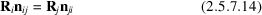forand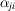. The discrepancy minimized in the method is the variance of the 3D structure that, by analogy to the 2D case (2.5.7.10)and (2.5.7.11)is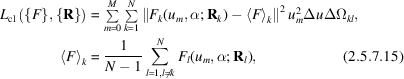where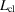is written in Fourier 3D polar coordinates and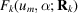is the Fourier transform of the kth projection in 2D polar coordinates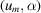with the orientation in 3D Fourier space given by the rotation matrix Rk. All Fourier planes Fk are considered to have zero thickness, so all discrepancies are calculated only along common lines and the partial average'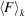is in fact an arrangement of N − 1 Fourier planes in 3D space. An approximation to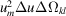is calculated by equating the values of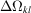to the areas of the Voronoi diagram cells constructed on a unit sphere for points of intersection of common lines with this unit sphere (see Section 2.5.6.6). Generally the method performs very well, particularly if the projection images cover 3D angular space evenly.

Some macromolecules, particularly those that have an elongated barrel-like shape, will have a strongly preferential orientation with respect to the direction of electron beam showing only what are often called side views', i.e., projections perpendicular to rotation along one axis corresponding to single-axis tilt data-collection geometry. These orthoaxial projections form single-axis tilt reconstruction geometry. In this case, Fourier transforms of all projections share only one common line, the line coinciding with the rotation axis, and clearly the common-lines-based method is not applicable to the ab initio structure determination. To cope with this situation, a method termed Sidewinder was developed (Pullan et al., 2006). It is based on the observation that a Fourier transform of a finite object with a diameter D can be considered to have a nonzero thickness 1/D (Fig. 2.5.6.5). Thus, if the angle between two central sections of the 3D Fourier transform of this object, as derived from 2D Fourier transforms of its projections, is not too large, then these two sections will share information in Fourier space that is proportional to the amount of overlap of the two slabs' in Fourier space. Using this observation, the general idea employed in Sidewinder is to calculate pairwise cross-correlation coefficients (CCCs) between class averages of side views and to use this information to deduce the values of the azimuthal Eulerian angles using the Monte Carlo minimization method (Fishman, 1995).

For structures that have reasonably high symmetry and for those for which it is possible to collect high-quality EM data, it is sometimes possible to determine the initial structure using the 3D projection alignment method, which will be described in the next section. However, the approach is extremely computationally intensive and it is virtually impossible to try the method repeatedly to verify that the approach converges to more-or-less the same 3D structure, as is recommended for other ab initio methods described in this section. When the method is successful it is quite powerful, as an intermediate resolution structure can be obtained without going through intermediate and quite laborious steps of analysis of the data. A word of caution is warranted: with the direct method, unless there is external evidence that the obtained structure is correct, it is possible to obtain a self-consistent but entirely incorrect model of the molecule.

In the absence of reliable objective measures of the correctness of the structure, one can apply common sense in order to spot definitely improbable 3D maps. Given the mass of the complex it is possible to calculate the corresponding volume, and thus the threshold at which the map should be examined (Section 2.5.7.11). If at this threshold the mass density is discontinuous or there are pieces of mass surrounding the structures, the map is most likely to be incorrect. Similarly, strong directional artifacts appearing as streaks permeating the structure indicate that either the collected projection images are dominated by one or two views of the structure or that the angular assignment is incorrect. In addition, the 3D map should be centred in the window box; although the centring is not strictly speaking a mathematical requirement for a successful reconstruction, all single-particle structure-determination software packages take advantage of the fact that for centred objects orientation searches are easier to perform. So, if the map is not centred it is a clear indication of the failure of the procedure. Finally, for symmetric structures there should be no large pieces of mass on the symmetry axes.

### References

Crowther, R. A., DeRosier, D. J. & Klug, A. (1970). The reconstruction of a three-dimensional structure from projections and its application to electron microscopy. Proc. R. Soc. London Ser. A, 317, 319–340.
Farrow, N. A. & Ottensmeyer, F. P. (1992). A posteriori determination of relative projection directions of arbitrarily oriented macromolecules. J. Opt. Soc. Am. A, 9, 1749–1760.
Fishman, G. (1995). Monte Carlo: Concepts, Algorithms, and Applications. New York: Springer.
Goncharov, A. B., Vainshtein, B. K., Ryskin, A. I. & Vagin, A. A. (1987). Three-dimensional reconstruction of arbitrarily oriented identical particles from their electron photomicrographs. Sov. Phys. Crystallogr. 32, 504–509.
Heel, M. van (1987a). Angular reconstitution: a posteriori assignment of projection directions for 3D reconstruction. Ultramicroscopy, 21, 111–124.
Heel, M. van, Gowen, B., Matadeen, R., Orlova, E. V., Finn, R., Pape, T., Cohen, D., Stark, H., Schmidt, R., Schatz, M. & Patwardhan, A. (2000). Single-particle electron cryo-microscopy: towards atomic resolution. Quart. Rev. Biophys. 33, 307–369.
Heel, M. van, Harauz, G. & Orlova, E. V. (1996). A new generation of the IMAGIC image processing system. J. Struct. Biol. 116, 17–24.
Penczek, P. A., Zhu, J. & Frank, J. (1996). A common-lines based method for determining orientations for N > 3 particle projections simultaneously. Ultramicroscopy, 63, 205–218.
Pullan, L., Mullapudi, S., Huang, Z., Baldwin, P. R., Chin, C., Sun, W., Tsujimoto, S., Kolodziej, S., Stoops, J. K., Lee, J. C., Waxham, M. N., Bean, A. J. & Penczek, P. A. (2006). The endosome-associated protein Hrs is hexameric and controls cargo sorting as a `master molecule'. Structure, 14, 661–671.